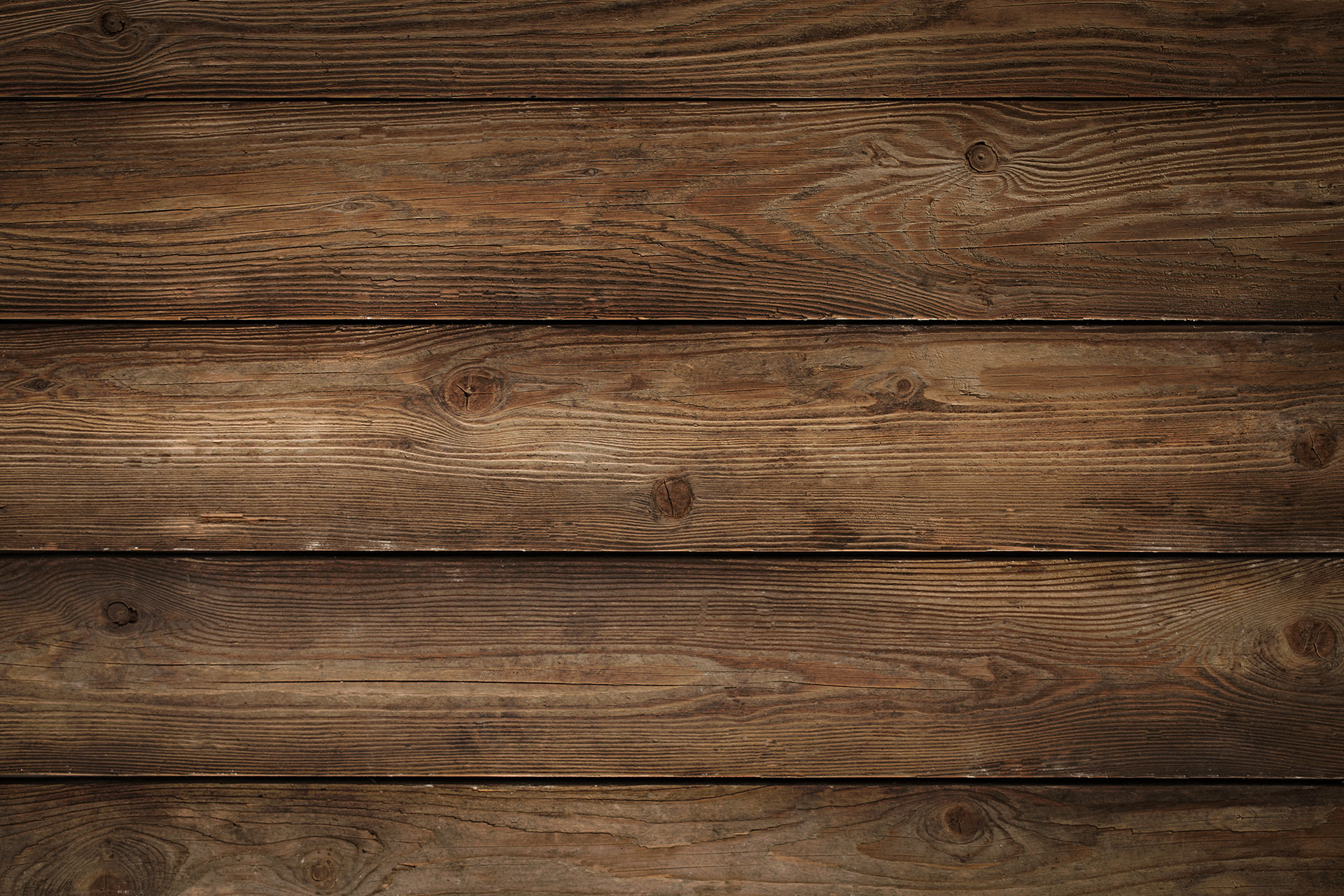top of pageSearch
• Ken Kraushaar

# Did you know? (#3)I thought I would cover a couple of conversions from metric to inches for ammunition and how shotgun gauges are determined.

Bullet Caliber Conversions:

The caliber of a bullet can be determined in either inches or millimeters. sometimes this can be confusing, but not so much once you look at it in terms of conversions.

1mm = approximately 4 caliber, (.393701 to be exact.) , but just use 4 and you'll find it easy to convert caliber from inches to millimeters, or vice versa.

the reason you would want to know this is because every popular caliber has a name in inches and a name in the metric system because that is what they use in Europe.

So...

.22 caliber (22 Div 4 = 5.5) = 5.56mm

.30 caliber (30 Div 4 = 7.5) = 7.62mm

.32 caliber (32 Div 4 = 8) = 7.92mm

.36 caliber (36 Div 4 = 9) = 9mm

.40 caliber (40 Div 4 = 10) = 10mm

.45 caliber (45 Div 4 = 11.25) = 11.25mm

and conversely........

5.56mm x 4 = .22 caliber

7.62mm x 4 = .30 caliber

7.65mm x 4 = .32 caliber

9mm x 4 = .36 caliber

10mm x 4 = .40 caliber

11.25mm x = .45 caliber

etc.

This is the basic way to determine the caliber of a bullet when converting from inches to millimeters or vice versa.

how shotgun shell gauges are determined:Say you're asked: "why does a 12 gauge shotgun have a bigger bore than a 20 gauge, when 12 is a smaller number?"

Until the 1840s there wasn't really an ability to measure a gun's bore with today's accuracy. Prior to that it was always possible to classify bores by the appropriate weight of the lead ball they took.

Keep in mind that before rifling, all guns were smooth bore, so after a gunsmith made a barrel he would also make a lead ball the same diameter of the bore of the barrel because you could fire it through the barrel as a load of shot. After making a number of lead balls of the same diameter as the bore, they were placed on the scale to see how many it took to make one pound.

for example:

How many lead balls which were 1/10th of a pound would it take to equal 1 pound?? in this case it would be 10, which would make the gun's bore a 10 gauge or back then you might call it a "10 bore"

So using this simple method, you can see how smaller lead balls, take more to equal 1 lb. so the gauge is the number of round lead balls fitting the bore required to make one pound.

example:

28 balls = 28 gauge

20 balls = 20 gauge

16 balls = 16 gauge

12 balls = 12 gauge.

the only exception to the rule is the .410 gauge. it's bore is measured in hundredths of an inch.

So, referring to the image above, the question would be, in the case of a 12 gauge, how many .727" balls would it take to equal 1lb? looking at a reference calculator for round ball weight found on the bear tooth bullets webpage, we find that a round ball of pure lead with a .727" diameter weighs approximately 579 grains. There are 7000 grains in a pound, so 7000/579 = 12.089810017271157167530224525043, or approximately 12 balls of .727" diameter lead balls., which gives us our gauge.

using the same calculator, we can look at a 20 gauge, which has a bore diameter of .617". using the same calculator, we find that a ball of pure lead with a dieameter of .617" weighs 354 grains. 7000/354= 19.774011299435028248587570621469 or rounded up is approximately 20 balls of pure lead. so there we have our 20 gauge.

now you know how shotgun gauges were determined!

Hopefully this little bit of info was useful. Stay safe and happy shooting!

44 views

See All
bottom of page